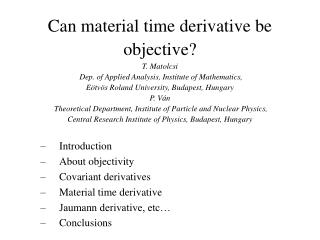DownloadDownload PresentationIntroduction About objectivity Covariant derivatives Material time derivative

# Introduction About objectivity Covariant derivatives Material time derivative

Download Presentation## Introduction About objectivity Covariant derivatives Material time derivative

- - - - - - - - - - - - - - - - - - - - - - - - - - - E N D - - - - - - - - - - - - - - - - - - - - - - - - - - -
##### Presentation Transcript

1. Can material time derivative be objective?T. Matolcsi Dep. of Applied Analysis, Institute of Mathematics, Eötvös Roland University, Budapest, HungaryP. Ván Theoretical Department, Institute of Particle and Nuclear Physics, Central Research Institute of Physics, Budapest, Hungary • Introduction • About objectivity • Covariant derivatives • Material time derivative • Jaumann derivative, etc… • Conclusions

2. Classical irreversible thermodynamics Local equilibrium (~ there is no microstructure) constitutive space Beyond local equilibrium: Nonlocality in space (structures) Nonlocality in time (memory and inertia)

3. ??? constitutive space (weakly nonlocal) Basic state space: a = (…..) Nonlocality in space (structures) Nonlocality in time (memory and inertia) Nonlocality in spacetime

4. Rheology Jaumann (1911) Oldroyd (1949, …) … Thermodynamic theory: Kluitenberg (1962, …), Kluitenberg, Ciancio and Restuccia (1978,…) Verhás (1977, …, 1998) Thermodynamic theory with co-rotational time derivatives. Experimental proof and prediction: - viscometric functions of shear hysteresis - instability of the flow !!

5. Material frame indifference • Noll (1958), Truesdell and Noll (1965) • Müller (1972, …) (kinetic theory) • Edelen and McLennan (1973) • Bampi and Morro (1980) • Ryskin (1985, …) • Lebon and Boukary (1988) • Massoudi (2002) (multiphase flow) • Speziale (1981, …, 1998), (turbulence) • Murdoch (1983, …, 2005) and Liu (2005) • Muschik (1977, …, 1998), Muschik and Restuccia (2002) • …….. Objectivity

6. About objectivity: Noll (1958) is a four dimensional objective vector, if where

7. is an objective four vector Spec. 1: Spec. 2: motion

8. If are inertial coordinates, the Christoffel symbol with respect to the coordinates has the form: Covariant derivatives: as the spacetime is flat there is a distinguished one. covector field mixed tensor field The coordinates of the covariant derivative of a vector field do not equal the partial derivatives of the vector field if the coordinatization is not linear.

9. where is the angular velocity of the observer

10. V(x) Ft(x) x t0 t is the point at time t of the integral curve V passing through x. Material time derivative: Flow generated by a vector field V. is the change of Φ along the integral curve.

11. V(x) is the covariant derivative of according to V. Ft(x) x t0 t Spec. 1: is a scalar substantial time derivative

12. Spec. 2: is a spacelike vector field The material time derivative of a vector – even if it is spacelike – is not given by the substantial time derivative.

13. for a spacelike vector Jaumann, upper convected, etc… derivatives: In our formalism: ad-hoc rules to eliminate the Christoffel symbols. For example: upper convected (contravariant) time derivative One can get similarly Jaumann, lower convected, etc…

14. Conclusions: • Objectivity has to be extended to a four dimensional setting. • Four dimensional covariant differentiation is fundamental in non-relativistic spacetime. The essential part of the Christoffel symbol is the angular velocity of the observer. • Partial derivatives are not objective. A number of problems arise from this fact. • Material time derivative can be defined uniquely. Its expression is different for fields of different tensorial order. space + time ≠ spacetime

15. Thank you for your attention.